# GMAT Data Sufficiency Strategies

Recently, in a GMAT forum, someone asked: “I am facing this problem with plugging numbers on the DS section. I realized this happens when I miss plugging in some specific numbers that just turn around the answer of the question. When I plug in, say, 2, the statement is sufficient, but plug in -1/2 and it’s not sufficient.  Please help me identify what is the best way to plug numbers:  is there a specific set of numbers I should test?”

In some ways, this is a very hard question to answer in the abstract, because often the nature of the question itself will dictate something about the choices we make.  Given that, here’s the best I can suggest for a rough–and–ready guide for what numbers to plug in, since number picking is a great GMAT Data Sufficiency strategy.:

1. First of all, it’s usually good to try 1, 0, and –1, if for no other reason than: they are usually very easy to plug in.

2. You almost always have to consider: a positive number greater than 1, a positive decimal less than 1, a negative number less than –1, and a negative decimal between zero and –1.

3. Notice that the quartet outlined in (b) is centered around zero.  If there’s some prominent algebraic factor that has a obvious root ––– e.g. the factor (x – 5) has a root at x = 5 –––– then it may well be pertinent to test both that root and a quartet analogous to the one in (b) centered around that root.  For example, if the factor (x – 5) were prominent, in the problem, then I would test the root x = 5 as well as x = 7, x = 5.1, x = 4.9, x = 3.

Now, that is a hyper–thorough list, and it would be very time–consuming to go through that entire procedure each and every time.  The nature of the problem often will allow you to streamline things.  For example, for any value that only appears in squared form, you don’t have to consider both positive and negative values, since both square to positive.

Also, remember: for Data Sufficiency questions, when you are trying to prove a statement insufficient, all you need are two possibilities – each one yields a different answer.  Once you have two different conclusions, and the statement is insufficient, you can stop picking.  It’s only when you suspect a statement is sufficient that you have to exhaust every possibility to try to disprove its sufficiency.

You want to give the statements a glance, so you don’t pick conditions that won’t be permitted by either statement, but after that, it’s often fruitful to play around with the prompt a bit, just enough to see if you can pick choices that indicate a conclusion each way.  Those choices will help you narrow things down in the statements, and you may even discover patterns that you can extend when applying the statements.  (If you had to sum up all of advanced mathematics in only three words, those three words most likely would be “extend known patterns.”  In other words, when you are extending a mathematical pattern, you are really thinking like a mathematician.)

Below are a couple DS problems, and in the solutions, I talk more specifically about the number–picking strategies I employ.

## Practice Problems: Data Sufficiency with Variables

1. Given that x ≠ 5, is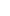>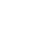(1) x > 0

(2) x > 10

(A) Statement (1) ALONE is sufficient, but statement (2) alone is not sufficient to answer the question asked.
(B) Statement (2) ALONE is sufficient, but statement (1) alone is not sufficient to answer the question asked.
(C) BOTH statements (1) and (2) TOGETHER are sufficient to answer the question asked, but NEITHER statement ALONE is sufficient to answer the question asked.=
(E) Statements (1) and (2) TOGETHER are NOT sufficient to answer the question asked, and additional data specific to the problem are needed.

2. Given that x ≠ 0, y ≠ 0,and (x + y) ≠ 0, is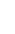>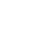(1) x > -1

(2) y > -1

(A) Statement (1) ALONE is sufficient, but statement (2) alone is not sufficient to answer the question asked.
(B) Statement (2) ALONE is sufficient, but statement (1) alone is not sufficient to answer the question asked.
(C) BOTH statements (1) and (2) TOGETHER are sufficient to answer the question asked, but NEITHER statement ALONE is sufficient to answer the question asked.
(E) Statements (1) and (2) TOGETHER are NOT sufficient to answer the question asked, and additional data specific to the problem are needed.

1. B

2. E

1. First of all, notice that one prominent factor in the prompt is (x – 5), and it’s squared.  The fact that (x – 5) is prominent makes us suspect that four possible choices should be

• 5 + (number bigger than one)
• 5 + (decimal between one and zero)
• 5 – (decimal between one and zero)
• 5 – (number bigger than one)

BUT, since the factor is squared, we don’t need the final two; when we subtract 5, they will result in negatives, which will duplicate the positives when squared.

This analysis suggests that x = 7 and x = 5.1 might be good numbers for starters.  Indeed, we find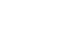which is smaller than 7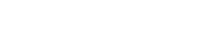which is larger than 5.1

Right there, two numbers lead to two different conclusions.  Any condition that allows us to plug in both 5.1 and 7 would be insufficient.

Also, notice: I am not as concerned with what would happen with negative values of x, because I’ve already scanned the answer choices and see that negatives values of x won’t be possible with either condition.  I will point out, though, that 1/(x – 5)^2 is something squared, so it will always be positive, and if x is negative, any positive number is always bigger than any negative number:<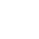for all negative values of x.

Statement #1: x > 0

This statement allows both x = 5.1 and x = 7, so we already know it’s insufficient.

Statement #2: x > 10

This statement doesn’t allow either x = 5.1 or x = 7.  We have to think about what’s possible now.  We know that when x = 10, it must be true that (x – 5) > 5.  The right side of the original inequality would become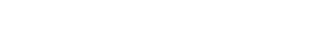We know that 1/25 is much smaller than one, and as the denominator gets bigger and bigger, those fractions will just get smaller and smaller.  They will always be smaller than one, so x > 1 >1/(number bigger than 25).  This statement is enough to conclude that x is always greater.  Statement #2 is sufficient.

2. Given that x ≠ 0 and (x + y) ≠ 0, is>Start with what’s true for the most obvious numbers.  Clearly, for both x and y equal to positive integers greater than one, this inequality is true: for x = 2 and y = 3,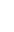>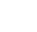= 2/5.   Making the denominator bigger makes the fraction smaller.

Strange things often happen with negatives and inequalities, so I’ll also try x = –2 and y = –3.  Negative over negative is positive, so this repeats the previous answer>, and the inequality is still true.

What is, of x and y, one is positive and one is negative? With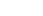and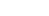, the right fraction is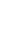, and the left fraction is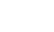, which is more negative, so now the inequality is false.

Notice that I didn’t even consider fractions, because, up until this point in the problem, I didn’t have to.  I was able to find different integer values that led to different conclusions.

Statement #1: x >–1

First of all, notice that our old friends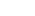and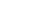are still valid choices, so the inequality is true for them.For the reverse, notice that we have already check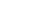and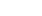, which is also permitted, and the inequality is false for that choice.  Two choices, two different conclusions.  Statement #1 by itself is insufficient.

Statement #2: y > –1

Again, our old friendsandare still valid choices, so the inequality is true for them.  Above, we also triedand, and the inequality is false for these.  Two choices, two different conclusions.  Statement #2 by itself is insufficient.

Combined Statements #1 and #2: x >–1 and y >–1

Yet again, our old friends x = 2 and y = 3 are still valid choices, so the inequality is true for them.  We need some new values, and the positive/negative mix seemed to be working well for us, so we will try: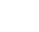and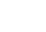,  which are still allowed under the combined condition.  Now,

left side ==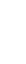=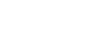=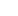right side ====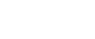=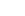These choices make the inequality false.  Two different choices for the variables, two different conclusions.  Even together, Statements #1 and #2 are insufficient.

•